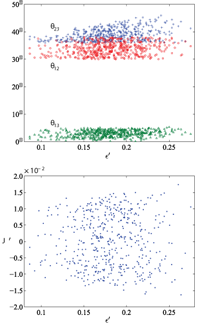## Non-Hermitian Perturbations to the Fritzsch Textures of Lepton and Quark Mass Matrices

Harald Fritzsch, Zhi-zhong Xing, Ye-Ling ZhouWe show that non-Hermitian and nearest-neighbor-interacting perturbations to the Fritzsch textures of lepton and quark mass matrices can make both of them fit current experimental data very well. In particular, we obtain \theta_{23} \simeq 45^\circ for the atmospheric neutrino mixing angle and predict \theta_{13} \simeq 3^\circ to 6^\circ for the smallest neutrino mixing angle when the perturbations in the lepton sector are at the 20% level. The same level of perturbations is required in the quark sector, where the Jarlskog invariant of CP violation is about 3.7 \times 10^{-5}. In comparison, the strength of leptonic CP violation is possible to reach about 1.5 \times 10^{-2} in neutrino oscillations.

http://arxiv.org/abs/1101.4272
High Energy Physics – Phenomenology (hep-ph)